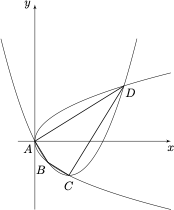# 每日一题构造与论证

A．四边形 $A B C D$ 不可能为平行四边形

B．存在四边形 $A B C D$，满足 $\angle A=\angle C$

C．若 $A B$ 过抛物线 $E$ 的焦点 $F$，则直线 $O A, O B$ 斜率之积恒为 $-2$

D．若 $\triangle OAC$ 为正三角形，则该三角形的面积为 $12\sqrt 3$